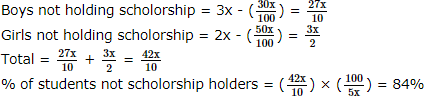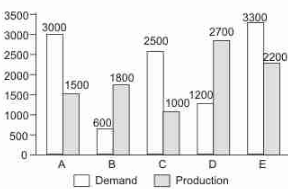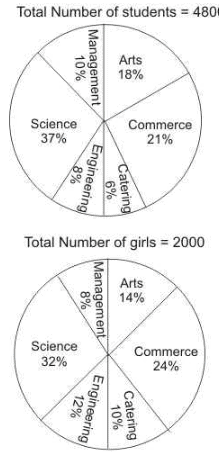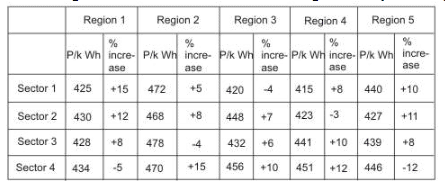Courses

# NABARD Manager Quant Aptitude Mock Test 5

## 20 Questions MCQ Test NABARD Manager - Mock Tests & Previous Year Papers | NABARD Manager Quant Aptitude Mock Test 5

Description
This mock test of NABARD Manager Quant Aptitude Mock Test 5 for Banking Exams helps you for every Banking Exams entrance exam. This contains 20 Multiple Choice Questions for Banking Exams NABARD Manager Quant Aptitude Mock Test 5 (mcq) to study with solutions a complete question bank. The solved questions answers in this NABARD Manager Quant Aptitude Mock Test 5 quiz give you a good mix of easy questions and tough questions. Banking Exams students definitely take this NABARD Manager Quant Aptitude Mock Test 5 exercise for a better result in the exam. You can find other NABARD Manager Quant Aptitude Mock Test 5 extra questions, long questions & short questions for Banking Exams on EduRev as well by searching above.
QUESTION: 1

### A father is 30 times older than his son. 18 years later, he will be only thrice as old as his son. Father's present age in years is

Solution:

F = 30 S
or, S = F/ 30
Also, F + 18 = 3(S + 18)
∴ F + 18 = 3( F/ 30 + 18)
or, F + 18 = F /10 + 54
or, F - F /10 = 36 or, 9 /10 F = 36
or, F = 36 x 10 /9 = 40 yrs

QUESTION: 2

### Rajneetha walks around the circular park in 15 minutes. If she walks at the rate of 5 km/hr, how much distance would she have to travel, at the minimum, to reach the centre of the park from any point on its perimeter?

Solution:

Time = 15 minutes
= 15 x 60 sec = 900 sec
Speed = 5 km/hr
= 5 x 5/ 18 m/sec
= 25 18 m/sec
Now Perimeter of the circular park = D
= S x T
= 25/ 18 x 900
2πR = 1250 metre
R = 1250 x 7 /2x 22 = 8750/ 44
= 198.86 metres
∴ Required minimum distance to reach the centre of the circle = 200 metre (approx)

QUESTION: 3

### What should come in place of the question-mark (?) in the following number series? 5690 5121 4552 3983 3414 2845?

Solution:
QUESTION: 4
The average number of students in five classes is 29. If the average number of students in class I,III and V is 30,then the total number of students in class II and IV is
Solution:
QUESTION: 5
The quantity of water in litres that must be added to 12 litres of beer priced at Rs 10 a litre to reduce the price to Rs 8 a litre is
Solution:
QUESTION: 6

The ratio of the number of boys and girls in a school is 3:2. 10% of the boys and 25% of the girls are scholarship holders. The percentage of the school students who are not scholarship holder is

Solution:

Let the boys = 3x
girls = 2xQUESTION: 7

An anti aircraft gun can fire four shots at a time. If the probabilities of the first, second, third and the last shot hitting the enemy aircraft are 0.7, 0.6, 0.5 and 0.4, what is the probability that four shots aimed at an enemy aircraft will bring the aircraft down?

Solution:

The enemy aircraft will be brought down even if one of the four shots hits the aircraft.
The opposite of this situation is that none of the four shots hit the aircraft.
The probability that none of the four shots hit the aircraft is given by:
(1 − 0.7)(1 − 0.6)(1 − 0.5)(1 − 0.4) = 0.3 x 0.4 x 0.5 x 0.6 = 0.036
So, the probability that at least one of the four hits the aircraft:
= 1 – 0.036 = 0.964

QUESTION: 8
A merchant bought an ivory box of Rs 4,321. What will be the approximate percentage of profit if he sold it for Rs 4,852?
Solution:
QUESTION: 9

If a:b=c:d=e:f=1:2, then (pa+qc+re):(pb+qd+rf) is equal to

Solution:

a : b = c : d = e : f = 1 : 2 = k
a = k, b = 2k, c = k, d = 2k, e = k, f = 2k
pa + qc + re = pk + qk + rk
= k (p + q + r)
pb + qd + rf = p x 2k + qx 2k + r x 2k
= 2k(p + q + r)
∴ (pa + qc + re) : (pb + qd + rf)
= k(p + q + r) : 2k(p + q + r)
= 1: 2

QUESTION: 10

Mr. Anuraag Awasthi deposits an amount of Rs 56500 to obtain a simple interest at the rate of 12 p.c.p.a. for 3 years. What total amout will Mr. Anurrag Awasthi get at the end of 3 years ?

Solution:

SI = P x R x T 100
⇒ SI = 56500 x 12 x 3 100
⇒ SI = Rs 20,340
⇒ Amount = Principle + SI
⇒ Amount = Rs (56500 + 20340) = Rs 76840

QUESTION: 11
Machine A can print one lakh books in 8 hrs. Machine B can do the same job in 10 hrs. Machine C can do the same job in 12 hrs. All the three machines start job at 9.00 a.m. Machine A breaks down at 11.00 a.m and other two machines finish the job. Approximately at what time will the job be finished?
Solution:
QUESTION: 12

How many metres of cloth 1.1m wide will be required to make a conical tent whose vertical height is 12m and base radius is 16m?

Solution:

Radius of cone, r = 16m
Height of cone, h = 12m
Slant height of cone = l = h 2 + r 2
= 12 2 + 16 2 = 144 + 256 = 400 = 20 m
Area of cloth required = Lateral surface area of the cone = πrl
= 22 7 x 16 x 20 = 7040 7 sq.m
Width of the cloth = 1.1m
∴ Length of the cloth = Area Width = 7040 x 10 7 x11 = 6400 7 = 914 2 7 metres

QUESTION: 13

In each of the following questions, two equations are given. You have to solve these equations and

2. I. x2 - 24x + 144 = 0
II. y2 - 26y + 169 = 0

Solution:

I. x2 - 24x + 144 = 0
⇒ x2 - 12x - 12x + 144 = 0
⇒ x(x - 12) - 12 (x - 12) = 0
⇒ (x - 12) (x - 12) = 0
⇒ x = 12
II. y2 - 26y + 169 = 0
⇒ (y - 13)2 = 0
⇒ y - 13 = 0
⇒ y = 13
x < y

QUESTION: 14

In each of the following questions, two equations are given. You have to solve these equations and

I. 2x2 + 3x - 20 = 0
II. 2y2 + 19y + 44 = 0

Solution:

I. 2x2 + 3x - 20 = 0
⇒ 2x2 + 8x - 5x - 20 = 0
⇒ 2x (x + 4) - 5 (x + 4) = 0
⇒ (x + 4) (2x - 5) = 0
⇒ x = -4 or 5252
II. 2y2 + 19y + 44 = 0
⇒ 2y2 + 11y + 8y + 44 = 0
⇒ y(2y + 11) + 4 (2y + 11) = 0
⇒ (y + 4) (2y + 11) = 0
⇒ y = -4 or -112112
x ≥ y

QUESTION: 15

These questions are based on the graph which shows the Demand and Production statistics of the five companies.What is the ratio of companies having more demand than production to those having more production than demand?

Solution:

A, C and E have more demand.
B and D have more production .
So the ratio is 3 : 2

QUESTION: 16

These questions are based on the graph which shows the Demand and Production statistics of the five companies.What is the difference between the average demand and the average production of five companies taken together ?

Solution:

Avg Demand = (3000 +600+2500+1200+3300)/5=10600/5 = 2120
Avg Production = 1840
So the Diff is 280

QUESTION: 17

Percentage distribution of candidates studying in various disciplines of a college and the percentage of girls in each discipline.
Percentage break up of student in each disciplineWhat is the number of girls studying in Engineering discipline ?

Solution:

Total number of girls studying in Engineering discipline
= 12% of total number of girls
= 2000 � 12/100 = 240

QUESTION: 18

Percentage distribution of candidates studying in various disciplines of a college and the percentage of girls in each discipline.
Percentage break up of student in each disciplineWhich discipline has the highest number of boys studying in it ?

Solution:

Number of boys in Science discipline
= 4800 � 37/100 - 2000 � 32/100
= 1776 - 640 = 1136
Number of boys in Commerce discipline
= 4800 � 21/100 - 2000 � 24/100
= 1008 - 480 = 528
Number of boys in Arts discipline
= 4800 � 18/100 - 2000 � 14/100
= 864 - 280 = 584
Number of boys in Management discipline
= 4800 � 10/100 - 2000 � 8/100
= 480 - 160 = 320
Hence, highest number of boys are studying in Science discipline.

QUESTION: 19

The following table gives the tariff [in paise per kilo-watt-hour (k Wh)] levied by the UPSEB in 1994-95, in four sectors and the regions within them. The table also gives the percentage change in the tariff as compared to 1991-92.The UPSEB supplies power under four categories; urban (25%), domestic (20%), industrial (40%), and rural (15%). In 1994-95, the total power produced by the UPSEB was 7875 megawatts.

If in 1994-95, there was a 10% decrease in the domestic consumption of powers as compared to that in 1991-92, what was the consumption of power in the rural sector in 1991-92?

Solution:

The domestic consumption in 1991-92 = 1,575/0.9 = 1,750 megawatts.
This constitutes 20% of total power consumed in 91-92 and the rural consumption constitutes 15% of total power in 91-92
Hence in 1991-92, the rural consumption = (1,750 � 15201520) = 1,312 megawatts

QUESTION: 20

The following table gives the tariff [in paise per kilo-watt-hour (k Wh)] levied by the UPSEB in 1994-95, in four sectors and the regions within them. The table also gives the percentage change in the tariff as compared to 1991-92.The UPSEB supplies power under four categories; urban (25%), domestic (20%), industrial (40%), and rural (15%). In 1994-95, the total power produced by the UPSEB was 7875 megawatts.

In the given two years, what is the total tariff paid by the urban sector ?

Solution:

The rates for the urban sector is not known